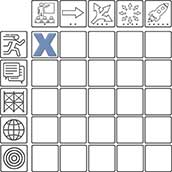Select Page

## Order of Operations

Active Learning | Entry Level

Subject: Math

##### Video Transcription

[Teacher voiceover]

Today what you’re going to see my class perform is order of operations, using the process of PEMDAS where they’ll be able to learn what process to do first, what’s the correct order, and what happens if they don’t follow the correct order.

Then there’s order of operations where you’re following parenthesis, then you go to exponents, then you do multiplication or division, whichever one comes first audition or subtraction, whichever one comes first, going left to right like you read a book.

[Teacher speaking to class]

We’re gonna start with order of operations. In order of operations, what we’re gonna do is you’re gonna tell me what my answer would be, and then you’re gonna tell me why that’s your answer.

First question, easy enough. Two plus two times two plus two. So two times two is four.

Okay, so what do I do now?

[Student 1 speaking]

[Teacher speaking to class]

[Student 1 speaking]

Left to right.

[Teacher speaking to class]

Left to right. So what’s four plus two and 6 plus 2?

[Student 1 speaking]

8

[Teacher speaking to class]

Do we agree with eight?

[Students]

Yes.

[Teacher speaking to class]

Okay, so following the order of operations, what happens if you don’t follow the right order?

[Students]

[Teacher speaking to class]

You get the wrong answer, good. Let’s try a new one. What can we do? Vanessa?

[Vanessa]

38.

[Teacher speaking to class]

38. Rudy?

[Rudy]

38.

[Teacher speaking to class]

38? Okay, so what is 3 2/3 power?

[Student 2 speaker]

27.

[Teacher speaking to student 2]

How did you get 27?

{Student 2}

3 times 3 times 3.

[Teacher speaking to class]

Good. 3 times 3 times times 3 is 27. Okay, so 27 plus 1 is 28, 28 plus 9 is 37… what’s wrong Mateo? It’s not 1? Why? So how do I do it? How do I divide? 2 over 1, what is 2 over 1? 2. Okay, so this should be 2. So now what do I do George? Is 29… is 38 .

##### Video Transcription

[Teacher voiceover]Today what you’re going to see my class perform is order of operations, using the process of PEMDAS where they’ll be able to learn what process to do first, what’s the correct order, and what happens if they don’t follow the correct order.Then there’s order of operations where you’re following parenthesis, then you go to exponents, then you do multiplication or division, whichever one comes first audition or subtraction, whichever one comes first, going left to right like you read a book.

[Teacher speaking to class]We’re gonna start with order of operations. In order of operations, what we’re gonna do is you’re gonna tell me what my answer would be, and then you’re gonna tell me why that’s your answer.First question, easy enough. Two plus two times two plus two. So two times two is four.

Okay, so what do I do now?

[Student 1 speaking]Add.[Teacher speaking to class]Add. Which way do I add?[Student 1 speaking]Left to right.[Teacher speaking to class]Left to right. So what’s four plus two and 6 plus 2?[Student 1 speaking]8[Teacher speaking to class]Do we agree with eight?[Students]Yes.[Teacher speaking to class]Okay, so following the order of operations, what happens if you don’t follow the right order?[Students]You get the wrong answer.[Teacher speaking to class]You get the wrong answer, good. Let’s try a new one. What can we do? Vanessa?[Vanessa]38.[Teacher speaking to class]38. Rudy?[Rudy]38.[Teacher speaking to class]38? Okay, so what is 3 2/3 power?[Student 2 speaker]27.[Teacher speaking to student 2]How did you get 27?{Student 2}

3 times 3 times 3.

[Teacher speaking to class]Good. 3 times 3 times times 3 is 27. Okay, so 27 plus 1 is 28, 28 plus 9 is 37… what’s wrong Mateo? It’s not 1? Why? So how do I do it? How do I divide? 2 over 1, what is 2 over 1? 2. Okay, so this should be 2. So now what do I do George? Is 29… is 38 .

Objectives

• Understand the order of PEMDAS.
• Apply PEMDAS to an equation to get the correct answer.
• Explain why the answer to an equation is correct using PEMDAS.

Procedure

• Students will solve problems using PEMDAS to recall the order of operations.

Technology Present

• Tablet
• Interactive white board
• Laptops with Internet access

Note: The TIM is about teaching, not technology. The placement of a lesson on the TIM is based entirely on the teaching practices demonstrated in the lesson and not on the specific technology employed. Effective pedagogy is our concern, not whether the tech involved is the latest, greatest, or most expensive. In fact, most of the sample videos were recorded some time ago as part of the original development of the Technology Integration Matrix. As you view these videos, focus on the teaching practices. When using the TIM-O to observe a lesson, remember that you can display the detailed indicators by clicking the tab on the right if you are completing a Matrix-based observation or are reviewing the TIM profile of a question-based lesson. The TIM Tools Admin Guide contains additional suggestions for observing a lesson and developing a TIM profile.

Video Reference Number: 1042Active Learning
• Entry:  LA   MA   SC   SS
• Adoption:  LA   MA   SC   SS
• Adaptation:  LA   MA   SC   SS
• Infusion:  LA   MA   SC   SS
• Transformation:  LA   MA   SC   SS
Collaborative Learning
• Entry:  LA   MA   SC   SS
• Adoption:  LA   MA   SC   SS
• Adaptation:  LA   MA   SC   SS
• Infusion:  LA   MA   SC   SS
• Transformation:  LA   MA   SC   SS
Constructive Learning
• Entry:  LA   MA   SC   SS
• Adoption:  LA   MA   SC   SS
• Adaptation:  LA   MA   SC   SS
• Infusion:  LA   MA   SC   SS
• Transformation:  LA   MA   SC   SS
Authentic Learning
• Entry:  LA   MA   SC   SS
• Adoption:  LA   MA   SC   SS
• Adaptation:  LA   MA   SC   SS
• Infusion:  LA   MA   SC   SS
• Transformation:  LA   MA   SC
Goal-Directed Learning
• Entry:  SS   LA   MA   SC   SS
• Adoption:  LA   MA   SC   SS
• Adaptation:  LA   MA   SC   SS
• Infusion:  LA   MA   SC   SS
• Transformation:  LA   MA   SC   SS